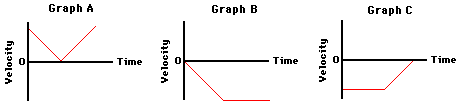# Lesson 1: Newton's First Law Of Motion

20 Questions | Attempts: 2123
ShareSettings• 1.
__________, a premier scientist in the seventeenth century, developed the concept of inertia.
• 2.
Imagine a place in the cosmos far from all gravitational and frictional influences. Suppose that you visit that place (just suppose) and throw a rock. The rock will
• A.

• B.

Continue in motion in the same direction at constant speed.

• C.

Will not move.

• D.

None of the above.

• 3.
A 2-kg object is moving horizontally with a speed of 4 m/s. How much net force is required to keep the object moving at this speed and in this direction?
• A.

0 N

• B.

1 N

• C.

10 N

• D.

None of the above.

• 4.
Mac and Tosh are arguing in the cafeteria. Mac says that if he flings the Jell-O with a greater speed it will have a greater inertia. Tosh argues that inertia does not depend upon speed, but rather upon mass. Who do you agree with?
• A.

Mac

• B.

Tosh

• C.

None of the above.

• 5.
Supposing you were in space in a weightless environment, would it require a force to set an object in motion?
• A.

Yes.

• B.

No.

• C.

None of the above.

• 6.
Fred spends most Sunday afternoons at rest on the sofa, watching pro football games and consuming large quantities of food. What effect (if any) does this practice have upon his inertia?
• A.

Fred's inertia stays the same!

• B.

Fred's inertia will decrease!

• C.

Fred's inertia will increase!

• D.

None of the above.

• 7.
Which one of the velocity-time graphs best describes the motion of the cat? Support your answer with sound reasoning.
• A.

Graph A

• B.

Graph B

• C.

Graph C

• D.

None of the above.

• 8.
Which one of the following dot diagrams best describes the motion of the falling cat from the time that they are dropped to the time that they hit the bottom of the pool? The arrows on the diagram represent the point at which the cat hits the water. Support your answer with sound reasoning.
• A.

Tape A

• B.

Tape B

• C.

Tape C

• D.

None of the above

• 9.
Several of Luke's friends were watching the motion of the falling cat. Being "physics types", they began discussing the motion and made the following comments. Indicate whether each of the comment(s) is correct or incorrect?
•  Once the cat hits the water, the forces are balanced and the cat will stop.
• A.

True

• B.

False

• 10.
Several of Luke's friends were watching the motion of the falling cat. Being "physics types", they began discussing the motion and made the following comments. Indicate whether each of the comment(s) is correct or incorrect?
• Upon hitting the water, the cat will accelerate upwards because the water applies an upward force.
• A.

True

• B.

False

• 11.
Several of Luke's friends were watching the motion of the falling cat. Being "physics types", they began discussing the motion and made the following comments. Indicate whether each of the comments is correct or incorrect?
• Upon hitting the water, the cat will bounce upwards due to the upward force.
• A.

True

• B.

False

• 12.
If the forces acting upon an object are balanced, then the object
• A.

Must not be moving.

• B.

Must be moving with a constant velocity.

• C.

Must not be accelerating.

• D.

None of these.

• 13.
A group of physics teachers is taking some time off for a little putt-putt golf. The 15th hole at the Hole-In-One Putt-Putt Golf Course has a large metal rim that putters must use to guide their ball towards the hole. Mr. S guides a golf ball around the metal rim When the ball leaves the rim, which path (1, 2, or 3) will the golf ball follow?
• A.

1

• B.

2

• C.

3

• D.

None of the above.

• 14.
A 4.0-kg object is moving across a friction-free surface with a constant velocity of 2 m/s. Which one of the following horizontal forces is necessary to maintain this state of motion?
• A.

0 N

• B.

5 N

• C.

10 N

• D.

None of the above.

• 15.
__________ is the tendency of an object to resist changes in its state of motion.
• 16.
_________ is the speed with a direction.
• 17.
______________ states that "An object at rest stays at rest and an object in motion stays in motion with the same speed and in the same direction unless acted upon by an unbalanced force."
• 18.
____________ is that quantity which depends solely upon inertia.
• 19.
An object is said to be acted upon by an unbalanced force only when there is an individual force that is not being balanced by a force of equal magnitude and in the opposite direction.
• A.

True

• B.

False

• 20.
To determine if the forces acting upon an object are balanced or unbalanced, an analysis must first be conducted to determine what forces are acting upon the object and in what direction.
• A.

True

• B.

False

## Related TopicsBack to top
×

Wait!
Here's an interesting quiz for you.